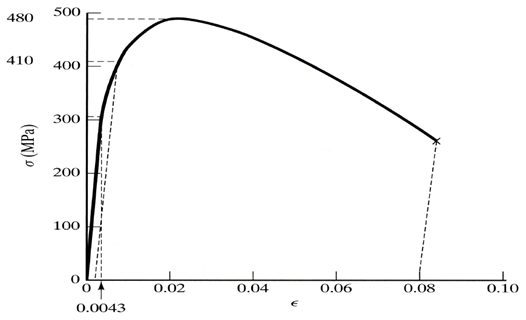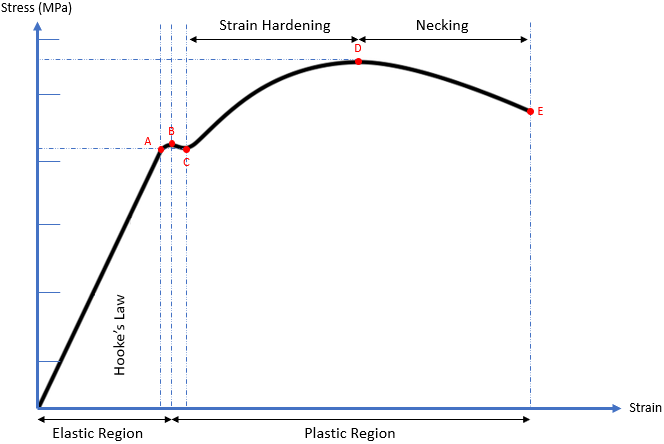## Aluminum Alloy Graphs

The plot below shows a stress-strain plot for tensile testing an aluminum alloy specimen. Determine the following parameters from this data:
1. What is the Elastic Modulus of this material?
2. What is the Tensile Strength of this alloy?
3. What is the Yield Strength of this alloy?
4. What is the ductility of this alloy?Hint
To find the Elastic Modulus, we need to calculate the slope (rise over run) of the initial loading.
Hint 2
Tensile Strength is the highest inflection point. The ductility is determined from the strain at unloading.
Breaking down the stress vs strain curve:From the origin to Point A, the ductile material is subjected to stress, resulting in a proportional strain. Young’s modulus (modulus of elasticity) is the slope (rise over run) of this section and is the proportionality constant that relates stress ( $$\sigma)$$ and strain ( $$\varepsilon$$ ). The straight line implies Hooke’s Law ( $$\sigma=E \varepsilon$$ ) is applicable.
From Points A to B, the slope becomes non-linear (Hooke’s Law is not applicable), but deformation is still elastic (the material will return to its original shape). As a result, Point A is known as the proportional limit and Point B is called the elastic point or the upper yield point (the max force a material can withstand elastically).
Additional stress beyond the elastic point will cause the material to enter the plastic region where the material will not return to its original shape after loading (like plastic materials). From Points B to C, both elastic and plastic deformation are occurring. It is not until Point C where the material is fully deforming plastically. Point C is called the lower yield point, and is the curve’s section associated with Yield strength.
When a material undergoes plasticity, the internal molecule structure experiences constant rearrangements. The material naturally resists this change by hardening (also known as strain hardening). As increased stress is applied from Points C to D, the material will elongate along its length (growing thinner and longer) until it reaches Point D, which is the material’s max strength. This section of the curve is associated with the material’s Ultimate (tensile) strength.
Continuing past the Ultimate strength, the material experiences necking where certain cross sectional areas are reducing/elongating locally. It is no longer uniform deformation across the entire material from Points D to E. The neck will grow weaker and thinner until it physically breaks apart at Point E. Point E is known as the fracture or rupture point.

To find the Elastic Modulus, we need to calculate the slope (rise over run) of the initial loading. Strain at the point of loss of linearity (~305 GPa) is the strain value 0.0043.
$$E=\frac{305MPa}{0.0043}=70.9\:GPa$$$Looking at the graph, the Tensile Strength is the highest inflection point. $$TS=480\:MPa$$$
Looking at the graph, the Yield Strength is: $$YS=410\:GPa$$
% $$EL=\frac{l_f-l_0}{l_0}\times 100=\varepsilon _{final} \times 100=(0.08)(100)=8$$ %